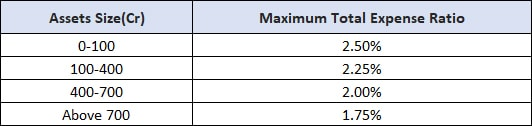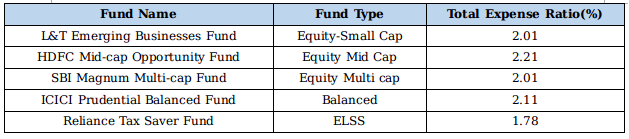Jun 15, 2018 2 min read

# Understanding the Basics of Total Expense Ratio

Read this blog to find out all about the expense ratio of mutual funds.
Nothing is free in this world and same goes for mutual fund schemes. Total Expense Ratio (TER) is a parameter which a number of investors overlook while choosing a fund.

It is at a later stage that they realize the true importance of expense ratio and how it can affect the returns they get on their investment. So, to avoid such things from happening, let's have a look at what Total Expense Ratio is and how it can affect your investment.

What Is Total Expense Ratio?

Total expense ratio as the name suggests is a measure that includes the total cost that is required to manage and operate a fund. These costs include expenses such as legal fees, management fees, registration fees, broker charges, fund managers salary, and other additional charges. The expense ratio can be calculated by dividing the total annual expenses with the total assets that were accumulated during that year.

Let's have a look at the regulations that SEBI has created regarding the expense ratio a fund can have. According to regulation 52 of SEBI, an AMC can charge a maximum of 2.50% TER and debt funds can charge a maximum of 2.25% total expense ratio. For the equity funds, below are the maximum TER for different asset sizes.How Can Expense Ratio Affect Your Returns?

The total expense ratio is a charge that you pay from your investment, so the amount that you pay as TER is the amount that is deducted from your investment which means, the more the expense ratio, the more the amount you pay in the form of reduced returns. Let's understand this with the help of an example.

Ram is a new investor and invested Rs 10,000 in a mutual fund scheme which has a total expense ratio of 2.5%. The scheme gave him a return of 5% in 1 year. Now, the total profit he will be making is the total return in that year minus the total expenses in that year, which equals 2.5%. So, the net profit for Ram will be Rs 250 and if the scheme gave no subsequent profit in that year, then this amount will be deducted from his investment.

Total Expense Ratio of Top Performing Mutual Funds

Now, let's have a look at the expense ratios of some of the top performing mutual fund schemes so that we can have a better understanding of TER.Conclusion:

There are several parameters that an investor should look at while choosing a mutual fund scheme and TER is one of them. Understanding these parameters will not only help you in understanding mutual fund market, but also in making sound investment decisions in the coming future.

For any kind of queries regarding mutual fund terminologies and mutual fund schemes, you can contact our experts at MySIPonline.